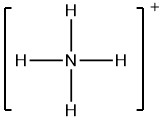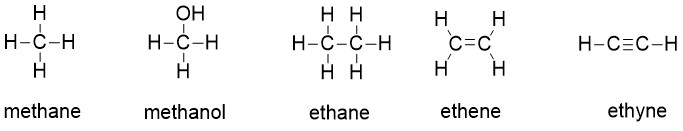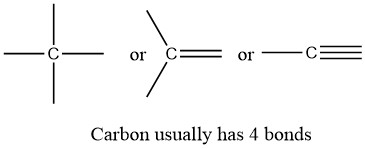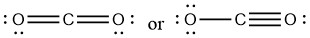# 2.2: Formal Charges

Objectives

After completing this section, you should be able to

1. calculate the formal charge of an atom in an organic molecule or ion.
2. identify and recognize the bonding patterns for atoms of carbon, hydrogen, oxygen, nitrogen and the halogens that have a formal charge of zero.

Key Terms

Make certain that you can define, and use in context, the key term below.

• valence electrons
• bonding and non-bonding electrons
• formal charge
• carbocations

Study Notes

It is more important that students learn to easily identify atoms that have formal charges of zero, than it is to actually calculate the formal charge of every atom in an organic compound.  Students will benefit by memorizing the "normal" number of bonds and non-bonding electrons around atoms whose formal charge is equal to zero.

A formal charge compares the number of electrons around a "neutral atom" (an atom not in a molecule) versus the number of electrons around an atom in a molecule.  Formal charge is assigned to an atom in a molecule by assuming that electrons in all chemical bonds are shared equally between atoms, regardless of relative electronegativity. To calculate formal charges, we assign electrons in the molecule to individual atoms according to these rules:• Non-bonding electrons are assigned to the atom on which they are located.
• Bonding electrons are divided equally between the two bonded atoms, so one electron from each bond goes to each atom.

The formal charge of each atom in a molecule can be calculated using the following equation:

Formal Charge = (# of valence electrons in free atom) - (# of lone-pair electrons) - (1/2 # of bond pair electrons)       Eqn. 2.3.1

To illustrate this method, let’s calculate the formal charge on the atoms in ammonia (NH3) whose Lewis structure is as follows:A neutral nitrogen atom has five valence electrons (it is in group 15). From the Lewis structure, the nitrogen atom in ammonia has one lone pair and three bonds with hydrogen atoms. Substituting into Equation 2.3.1, we obtain

Formal Charge of N = (5 valence e-) - (2 lone pair e-) - (1/2 x 6 bond pair e-) = 0

A neutral hydrogen atom has one valence electron. Each hydrogen atom in the molecule has no non-bonding electrons and one bond. Using Equation 2.3.1 to calculate the formal charge on hydrogen, we obtain

Formal Charge of H = (1 valence e-) - (0 lone pair e-) - (1/2 x 2 bond pair e-) = 0

The sum of the formal charges of each atom must be equal to the overall charge of the molecule or ion.  In this example, the nitrogen and each hydrogen has a formal charge of zero. When summed the overall charge is zero, which is consistent with the overall neutral charge of the NH3 molecule.Typically, the structure with the most formal charges of zero on atoms is the more stable Lewis structure. In cases where there MUST be positive or negative formal charges on various atoms, the most stable structures generally have negative formal charges on the more electronegative atoms and positive formal charges on the less electronegative atoms. The next example further demonstrates how to calculate formal charges for polyatomic ions.

Example 2.3.1

Calculate the formal charges on each atom in the NH4+ ion.

Given: chemical species

Strategy:

Identify the number of valence electrons in each atom in the NH4+ ion. Use the Lewis electron structure of NH4+ to identify the number of bonding and non-bonding electrons associated with each atom and then use Equation 2.3.1 to calculate the formal charge on each atom.

Solution:

The Lewis electron structure for the NH4+ ion is as follows:The nitrogen atom in ammonium has zero non-bonding electrons and 4 bonds.  Using Equation 2.3.1, the formal charge on the nitrogen atom is therefore

Formal Charge of N = (5 valence e-) - (0 lone pair e-) - (1/2 x 8 bond pair e-) = +1

formalcharge(N)=5(0+82)=0

Each hydrogen atom in has one bond and zero non-bonding electrons. The formal charge on each hydrogen atom is therefore

Formal Charge of H = (1 valence e-) - (0 lone pair e-) - (1/2 x 2 bond pair e-) = 0

formalcharge(H)=1(0+22)=0

The formal charges on the atoms in the NH4+ ion are thusAdding together the formal charges on the atoms should give us the total charge on the molecule or ion. In this case, the sum of the formal charges is 0 + 1 + 0 + 0 + 0 = +1, which is the same a s the overall charge of the ammonium polyatomic ion.

Exercise $$\PageIndex{1}$$

Write the formal charges on all atoms in BH4.

.### Common bonding patterns in organic structures

The calculation method reviewed above for determining formal charges on atoms is an essential starting point for a novice organic chemist, and works well when dealing with small structures. But this method becomes unreasonably time-consuming when dealing with larger structures. It would be exceptionally tedious to determine the formal charges on each atom in 2'-deoxycytidine (one of the four nucleoside building blocks that make up DNA) using equation 2.3.1.And yet, organic chemists, and especially organic chemists dealing with biological molecules, are expected to draw the structure of large molecules such as this on a regular basis. Clearly, you need to develop the ability to quickly and efficiently draw large structures and determine formal charges. Fortunately, this only requires some practice with recognizing common bonding patterns.

### Carbon

Carbon, the most important element for organic chemists. In the structures of methane, methanol, ethane, ethene, and ethyne, there are four bonds to the carbon atom.  And each carbon atom has a formal charge of zero.  In other words, carbon is tetravalent, meaning that it commonly forms four bonds.Carbon is tetravalent in most organic molecules, but there are exceptions.  Later in this chapter and throughout this book are examples of organic ions called ‘carbocations’ and carbanions’, in which a carbon atom has a positive or negative formal charge, respectively. Carbocations occur when a carbon has only three bonds and no lone pairs of electrons.  Carbocations have only 6 valence electrons and a formal charge of +1.  Carbanions occur when the carbon atom has three bonds plus one lone pair of electrons. Carbanions have 8 valence electrons and a formal charge of -1.Two other possibilities are carbpon radicals and carbenes, both of which have a formal charge of zero. A carbon radical has three bonds and a single, unpaired electron.  Carbon radicals have 7 valence electrons and a formal charge of zero. Carbenes are a highly reactive species, in which a carbon atom has two bonds and one lone pair of electrons, giving it a formal charge of zero. You may encounter carbenes in more advanced chemistry courses, but they will not be discussed any further in this book.

You should certainly use the methods you have learned to check that these formal charges are correct for the examples given above. More importantly, you will need, before you progress much further in your study of organic chemistry, to simply recognize these patterns (and the patterns described below for other atoms) and be able to identify carbons that bear positive and negative formal charges by a quick inspection.

### Hydrogen

The common bonding pattern for hydrogen is easy: hydrogen atoms in organic molecules typically have only one bond, no unpaired electrons and a formal charge of zero. The exceptions to this rule are the proton, H+, the hydride ion, H-, and the hydrogen radical, H..  The proton is a hydrogen with no bonds and no lone pairs and a formal charge of +1.  The hydride ion is a is a hydrogen with no bonds, a pair of electrons, and a formal charge of -1.  The hydrogen radical is a hydrogen atom with no bonds, a single unpaired electron and a formal charge of 0. Because this book concentrates on organic chemistry as applied to living things, however, we will not be seeing ‘naked’ protons and hydrides as such, because they are too reactive to be present in that form in aqueous solution. Nonetheless, the idea of a proton will be very important when we discuss acid-base chemistry, and the idea of a hydride ion will become very important much later in the book when we discuss organic oxidation and reduction reactions. As a rule, though, all hydrogen atoms in organic molecules have one bond, and no formal charge.

### Oxygen

The common arrangement of oxygen that has a formal charge of zero is when the oxygen atom has 2 bonds and 2 lone pairs.   Other arrangements are oxygen with 1 bond and 3 lone pairs, that has a -1 formal charge, and oxygen with 3 bonds and 1 lone pair that has a formal charge of +1.  All three patterns of oxygen fulfill the octet rule.If it has two bonds and two lone pairs, as in water, it will have a formal charge of zero. If it has one bond and three lone pairs, as in hydroxide ion, it will have a formal charge of-1. If it has three bonds and one lone pair, as in hydronium ion, it will have a formal charge of +1.

When we get to our discussion of free radical chemistry in chapter 17, we will see other possibilities, such as where an oxygen atom has one bond, one lone pair, and one unpaired (free radical) electron, giving it a formal charge of zero. For now, however, concentrate on the three main non-radical examples, as these will account for virtually everything we see until chapter 17.

### Nitrogen

Nitrogen has two major bonding patterns, both of which fulfill the octet rule:If a nitrogen has three bonds and a lone pair, it has a formal charge of zero. If it has four bonds (and no lone pair), it has a formal charge of +1. In a fairly uncommon bonding pattern, negatively charged nitrogen has two bonds and two lone pairs.

### Phosphorus and Sulfur

Two third row elements are commonly found in biological organic molecules: phosphorus and sulfur. Although both of these elements have other bonding patterns that are relevant in laboratory chemistry, in a biological context sulfur almost always follows the same bonding/formal charge pattern as oxygen, while phosphorus is present in the form of phosphate ion (PO43-), where it has five bonds (almost always to oxygen), no lone pairs, and a formal charge of zero. Remember that elements in the third row of the periodic table have d orbitals in their valence shell as well as s and p orbitals, and thus are not bound by the octet rule.### Halogens

The halogens (fluorine, chlorine, bromine, and iodine) are very important in laboratory and medicinal organic chemistry, but less common in naturally occurring organic molecules. Halogens in organic compounds usually are seen with one bond, three lone pairs, and a formal charge of zero. Sometimes, especially in the case of bromine, we will encounter reactive species in which the halogen has two bonds (usually in a three-membered ring), two lone pairs, and a formal charge of +1.These rules, if learned and internalized so that you don’t even need to think about them, will allow you to draw large organic structures, complete with formal charges, quite quickly.

Once you have gotten the hang of drawing Lewis structures, it is not always necessary to draw lone pairs on heteroatoms, as you can assume that the proper number of electrons are present around each atom to match the indicated formal charge (or lack thereof). Occasionally, though, lone pairs are drawn if doing so helps to make an explanation more clear.

## Using Formal Charges to Distinguish between Lewis StructuresAs an example of how formal charges can be used to determine the most stable Lewis structure for a substance, we can compare two possible structures for CO2. Both structures conform to the rules for Lewis electron structures.## CO2

1. C is less electronegative than O, so it is the central atom.2. C has 4 valence electrons and each O has 6 valence electrons, for a total of 16 valence electrons.3. Placing one electron pair between the C and each O gives O–C–O, with 12 electrons left over.4. Dividing the remaining electrons between the O atoms gives three lone pairs on each atom:This structure has an octet of electrons around each O atom but only 4 electrons around the C atom.

5. No electrons are left for the central atom.6. To give the carbon atom an octet of electrons, we can convert two of the lone pairs on the oxygen atoms to bonding electron pairs. There are, however, two ways to do this. We can either take one electron pair from each oxygen to form a symmetrical structure or take both electron pairs from a single oxygen atom to give an asymmetrical structure:Both Lewis electron structures give all three atoms an octet. How do we decide between these two possibilities? The formal charges for the two Lewis electron structures of CO2 are as follows:

Both Lewis structures have a net formal charge of zero, but the structure on the right has a +1 charge on the more electronegative atom (O). Thus the symmetrical Lewis structure on the left is predicted to be more stable, and it is, in fact, the structure observed experimentally. Remember, though, that formal charges do not represent the actual charges on atoms in a molecule or ion. They are used simply as a bookkeeping method for predicting the most stable Lewis structure for a compound.Note

The Lewis structure with the set of formal charges closest to zero is usually the most stable.Example 2.3.2

The thiocyanate ion (SCN), which is used in printing and as a corrosion inhibitor against acidic gases, has at least two possible Lewis electron structures. Draw two possible structures, assign formal charges on all atoms in both, and decide which is the preferred arrangement of electrons.

Given: chemical species

Asked for: Lewis electron structures, formal charges, and preferred arrangement

Strategy:

A Use the step-by-step procedure to write two plausible Lewis electron structures for SCN.

B Calculate the formal charge on each atom using Equation 2.3.1.

C Predict which structure is preferred based on the formal charge on each atom and its electronegativity relative to the other atoms present.

Solution:

A Possible Lewis structures for the SCN ion are as follows:

B We must calculate the formal charges on each atom to identify the more stable structure. If we begin with carbon, we notice that the carbon atom in each of these structures shares four bonding pairs, the number of bonds typical for carbon, so it has a formal charge of zero. Continuing with sulfur, we observe that in (a) the sulfur atom shares one bonding pair and has three lone pairs and has a total of six valence electrons. The formal charge on the sulfur atom is therefore 6 - (6 + 2/2) = -1. In (b), the sulfur atom has a formal charge of 0. In (c), the sulfur atom has a formal charge of +1. Continuing with the nitrogen, we observe that in (a) the nitrogen atom shares three bonding pairs and has one lone pair and has a total of 5 valence electrons. The formal charge on the nitrogen atom is therefore 5 - (2 + 6/2) = 0. In (b), the nitrogen atom has a formal charge of -1. In (c), the nitrogen atom has a formal charge of -2.

C Which structure is preferred? Structure (b) is preferred because the negative charge is on the more electronegative atom (N), and it has lower formal charges on each atom as compared to structure (c): 0, −1 versus +1, −2.

Exercise $$\PageIndex{2}$$

Salts containing the fulminate ion (CNO) are used in explosive detonators. Draw three Lewis electron structures for CNO and use formal charges to predict which is more stable. (Note: N is the central atom.)The second structure is predicted to be the most stable.

### Exercises

1. Draw the Lewis structure of each of the molecules listed below.

CH3+, NH2, CH3, NH4+, BF4

In each case, use the method of calculating formal charge described to satisfy yourself that the structures you have drawn do in fact carry the charges shown.

1.#### Questions

Q2.3.1

Give the formal charges for all non-hydrogen atoms in the following moelcules:

BH4, H2O, CH3O

#### Solutions

S2.3.1

BH4 (B = −1)

H2O (O = 0)

CH3O (C = 0, O = −1)